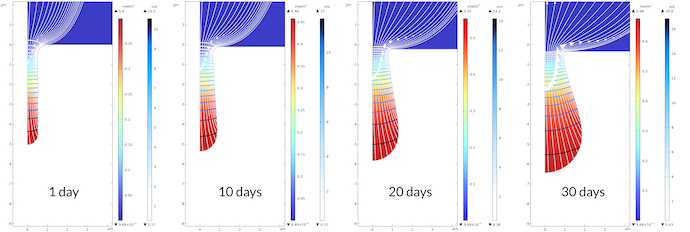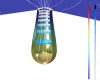# 在 COMSOL Multiphysics® 中模拟点蚀

2021年 7月 13日

### 腐蚀过程简介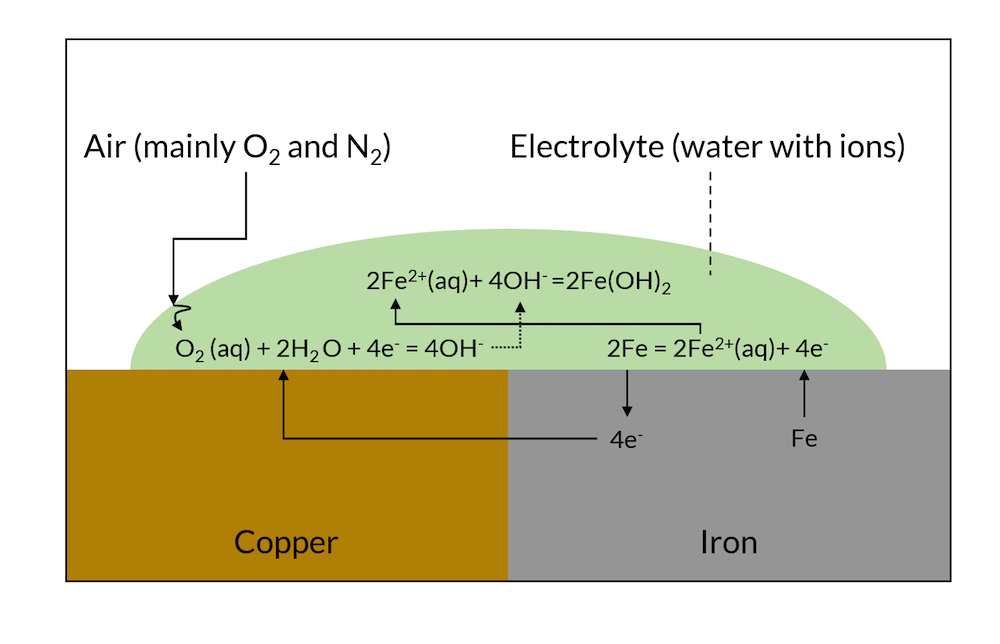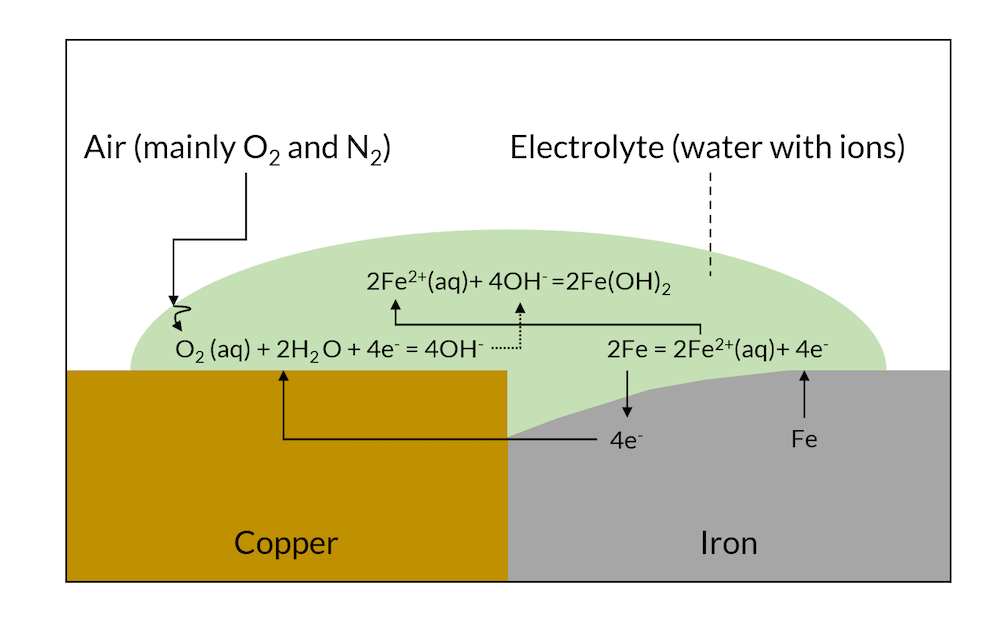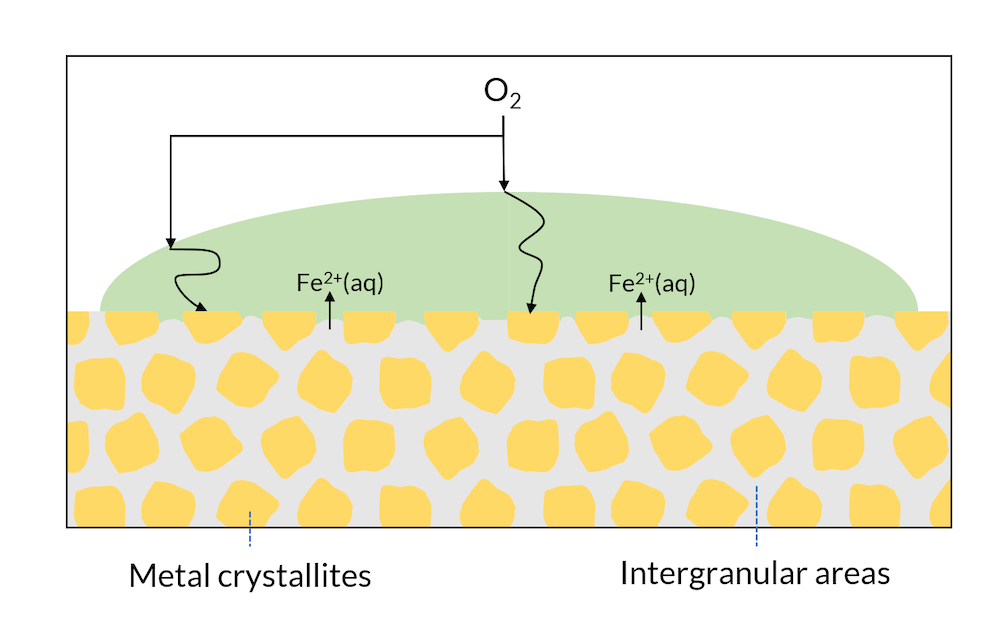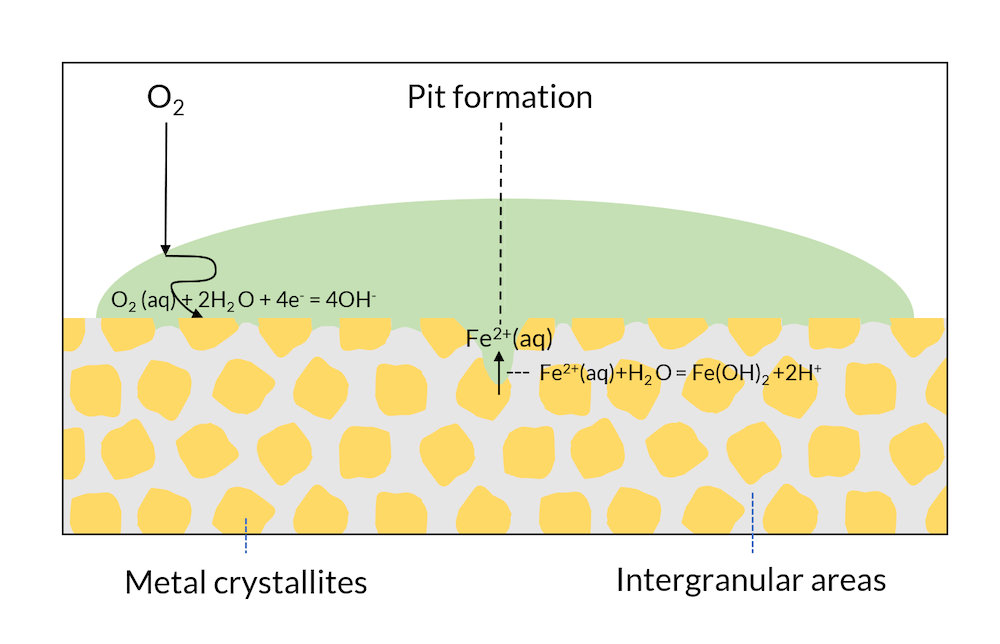### 模拟点蚀

• 铁在阳极极化表面溶解：$2Fe = 2F{e^{2 + }}\left( {aq} \right) + 4{e^ – }$
• 电解液中的沉积反应：$F{e^{2 + }}\left( {aq} \right) + 2O{H^ – } = Fe{\left( {OH} \right)_2}\left( s \right)$
• 电解液中水的电离：${H_2}O = {H^ + } + O{H^ – }$

• $F{e^{2 + }}$
• ${H^ + }$
• $O{H^ – }$
• $N{a^ + }$
• $C{l^ – }$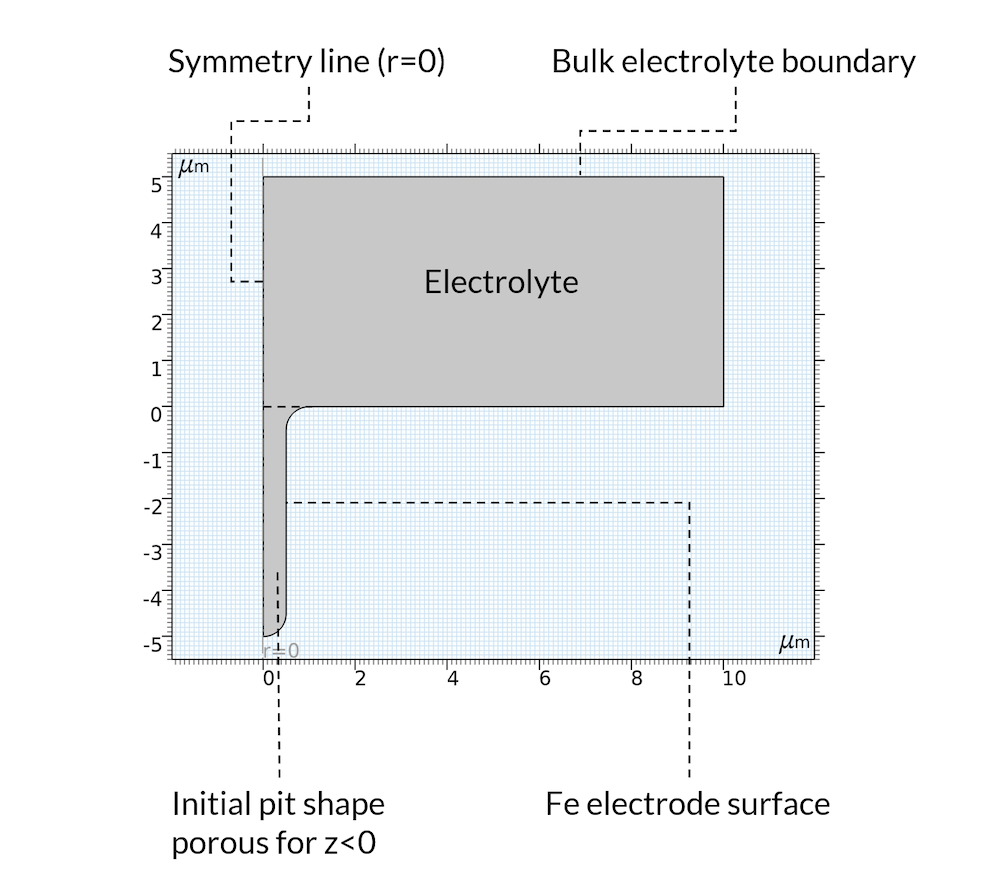### 结果